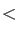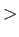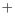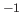### Keyword FREQUENCY

This keyword activates the frequency analysis.
Options:
 FIXED A partial frequency analysis is requested. RAMAN The Raman intensities are calculated. RESTART The frequency analysis is restarted from the deMon.rst file. VIB=RealScaling factor for the numerical step size.
Description:
By default, the infra-red intensities of the vibrations are calculated. With the option RAMAN, the Raman intensities (in atomic units) and the depolarization ratio  are calculated also. This is considerably more time-consuming than a standard frequency analysis. The RESTART option permits the restart of a frequency analysis. The Hessian matrix elements already calculated are read from the restart file deMon.rst and the analysis is continued. The numerical step size can be scaled with the VIB option. By default, the step size is 0.005 a.u. for all theoretical models. With VIB=2 or VIB=0.2, this step size can be scaled to 0.01 a.u. or 0.001 a.u., respectively. Note that numerical frequency calculations can be sensitive to the SCF convergence. To improve the SCF convergence in the finite-step SCF calculations the options TOL and CDF of the SCFTYPE keyword (see 4.5.1) can be used.

The FIXED option specifies a partial frequency analysis, that is, calculation of frequencies involving only the motion of certain atoms. The element or atomic symbols of the atoms that are to be treated in the frequency analysis must be specified in the keyword body of the FREQUENCY keyword. The following example input shows a frequency analysis for the CO in the CuCOcation.

``` CHARGE +1
FREQUENCIES FIXED
C O
#
GEOMETRY Z-MATRIX ANGSTROM
Cu
C   Cu  R1
O   C   R2  Cu  180.0
#
VARIABLES
R1  1.779932
R2  1.136288
```

The calculated CO stretching frequency of 2250 cmis in excellent agreement with the result from an unconstrained frequency analysis (2251 cm).